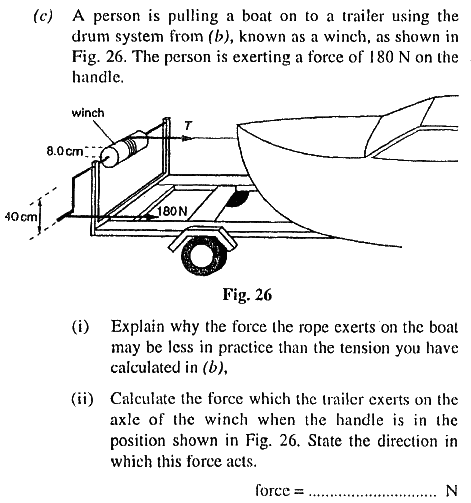# Question of Force - why it differs in reality?

• PhysicStud01
In summary, the question discusses the difference between an ideal winch and a real winch, as well as the reason for a specific answer to a problem involving a winch. It explains that the tension on the rope is not the only force acting on the drum/axle, and that there must be another force acting to the left for the sum of all forces to be zero. The conversation also touches on the concept of torque and how it relates to rotational motion, and the mechanical advantage offered by different drum sizes on a winch. Finally, it addresses the question of how a trailer exerts a force on the axle, and explains that in order for the axle to remain in place, there must be a horizontal force to the

## Homework Statement## The Attempt at a Solution

could you give me the reason for part i. Can't figure the reason
for the second part. it says ans is 1080N to left. shouldn't it be 900N. and why is it to the left?

What's the difference between an ideal winch and a real winch?

for the second part. it says ans is 1080N to left. shouldn't it be 900N. and why is it to the left?

The tension T is 900N but that's not the only force on the drum/axle.

The tension and one other force act to the right. If the drum/axle doesn't accelerate to the right there must be another force acting to the left so that the sum of all forces on the drum/axle is zero.

CWatters said:
What's the difference between an ideal winch and a real winch?
is work done as friction. what exactly would be the wasted energy here?
CWatters said:
The tension T is 900N but that's not the only force on the drum/axle.

The tension and one other force act to the right. If the drum/axle doesn't accelerate to the right there must be another force acting to the left so that the sum of all forces on the drum/axle is zero.
if it's the reaction of 180N, won't it be on the axle itself, but how does the trailer exert this force. the axle is in the air?

CWatters said:
The tension T is 900N but that's not the only force on the drum/axle.

The tension and one other force act to the right. If the drum/axle doesn't accelerate to the right there must be another force acting to the left so that the sum of all forces on the drum/axle is zero.
another thing. by calculation, i went as follows, Tx0.08 = 180x0.4 giving T = 900N
why it don't understand why it's this way. isn't there actually a resultant moment there. so, why should we equate the moments. if it was force, then I understand from Newton's 3rd law, its a reaction. but why is it this way here? i only got the numerical value without understanding what's really happening and why? could you explain please.

PhysicStud01 said:
if it's the reaction of 180N, won't it be on the axle itself, but how does the trailer exert this force. the axle is in the air?
The vertical supports holding the axle are part of the trailer. If the axle is to remain in place, there must be a horizontal force to the left balancing the sum of horizontal forces to the right.

PhysicStud01 said:
another thing. by calculation, i went as follows, Tx0.08 = 180x0.4 giving T = 900N
That's the tension in the rope.
so, why should we equate the moments. if it was force, then I understand from Newton's 3rd law, its a reaction. but why is it this way here? i only got the numerical value without understanding what's really happening and why? could you explain please.
The winch's handle length vs. drum radius offer a mechanical advantage. A narrower drum means the handle will be easier for you to turn, but you'll have to turn it more times to draw in the same length of rope.

NascentOxygen said:
That's the tension in the rope.

The winch's handle length vs. drum radius offer a mechanical advantage. A narrower drum means the handle will be easier for you to turn, but you'll have to turn it more times to draw in the same length of rope.
yeah, but why equate the moment. shoudl there be a resultant moment, for the drum to rotate

PhysicStud01 said:
is work done as friction. what exactly would be the wasted energy here?

Nothing to do with energy... The axle is subject to three torques that sum to zero if it's rotating at constant (angular) velocity. The input torque, the output torque and the torque due to friction.

yeah, but why equate the moment. shoudl there be a resultant moment, for the drum to rotate

No. If you assume the drum is revolving at constant (angular) velocity then it's not accelerating and the torques must sum to zero.

if it's the reaction of 180N, won't it be on the axle itself, but how does the trailer exert this force. the axle is in the air?

Via the bearing.

If it's not accelerating the linear forces sum to zero.

Last edited:
If the boat is being drawn in at a steady speed, you can consider that an equilibrium, so sum of forces is zero.

Might help if you can see the similarity between...

Force = mass * acceleration
and
Torque = moment of inertia * angular acceleration